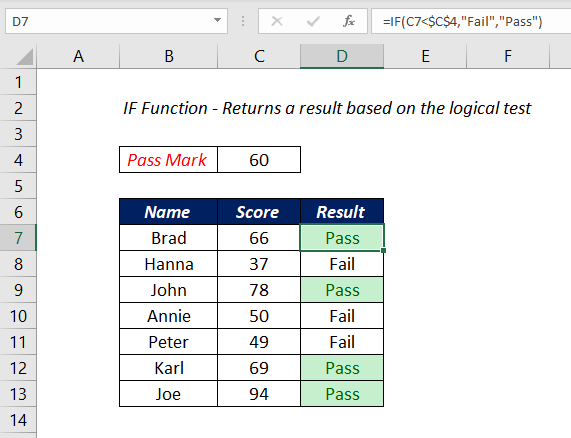# IF FunctionThe Excel IF function is used to perform a logical test and return a value based on the test result. One value for TRUE result and another value for FALSE result.

Logical

### Purpose

Test a specific condition

### Return value

One of the two values supplied for TRUE or FALSE

### Syntax

`=IF(logical_test,[value_if_true],[value_if_false])`

### Arguments

logical_test – A logical expression that can be evaluated as TRUE or FALSE

value_if_true – [optional] The value to return when the logical_test evaluates to TRUE

value_if_false – [optional] The value to return when the logical_test evaluates to FALSE

### Examples

#### Check the score of a candidate and return PASS or FAIL on the basis of the score value

In this example, IF function is used to check whether the score of the candidate is Greater than or Less than 60 and based on the test result, return the text “PASS” or “FAIL“.

`=IF(C3>60,"PASS","FAIL")`

The literal translation of the above formula is ‘If the value in the cell C3 is greater 60, return the text PASS, otherwise return the text FAIL’.

The logical flow of this test can be reversed and the same result can be obtained. i.e. instead of checking whether the score is greater than 60 and return PASS or FAIL, the formula will check whether the score is less than 60 and return FAIL or PASS.

`=IF(C3<60,"FAIL","PASS")`
`=IF(C3>60,"PASS","FAIL") is same as =IF(C3<60,"FAIL","PASS")`

In the above example, we have hardcoded the pass mark, i.e. the value 60 into the formula. Now, we will use a cell reference C2 that contains the pass mark, inside the IF function.

`=IF(C5>\$C\$2,"PASS","FAIL")`

here the formula checks whether the value in the cell C5 is greater than the value in the cell C2 and return PASS or FAIL based on the result.

#### Arithmetic operations using IF Function

In the following example, IF function is used to find out the non-negative difference between values in columns A and B. The formulas A-B or B-A may sometimes return a negative value. But the following formula using IF function will always return a positive value.

`=IF(B3>C3,B3-C3,C3-B3)`

The literal translation of the above formula is ‘If the value in the cell B3 is greater than the value in the cell C3, subtract the value in the cell C3 from that in the cell B3 and return that value, otherwise subtract the value in the cell B3 from that in the cell C3 and return that value.

One more example where IF function is used to compare two values and perform multiplication or division between them.

`=IF(B3>C3,B3/C3,C3*B3)`

The function will check whether the value in the cell B3 is greater than the value in the cell C3. If the value in B3 is greater than the value in the cell C3, the value in B3 is divided by the value in C3 and the function will return that result. If the value in the B3 is not greater than the value in the cell C3, the function will return the product of values in B3 and C3.

### NESTED IFs

When an IF function is used within another IF function, that technique is called Nesting of IFs. This is usually done to check multiple conditions.

Following is an example in which the IF function is used to check a score and return different grades based on the score value.

`=IF(C3<70,"D",IF(C3<=80,"C",IF(C3<=90,"B","A")))`

The above formula when broken down into steps, will read like the following

Step 1: Check whether the value in the cell C3 is less than 70. If so, return the text D otherwise, go to Step 2 (Second IF function)

Step 2: Check whether the value in the cell C3 is less than 80. If so return the text C otherwise, go to Step 3 (Third IF Function)

Step 3: Check whether the value in the cell C3 is less than 90. If so return the text B otherwise return A.

## Logical Operators for IF Function

The following are the list of operators that can be used inside IF function to perform logical tests.